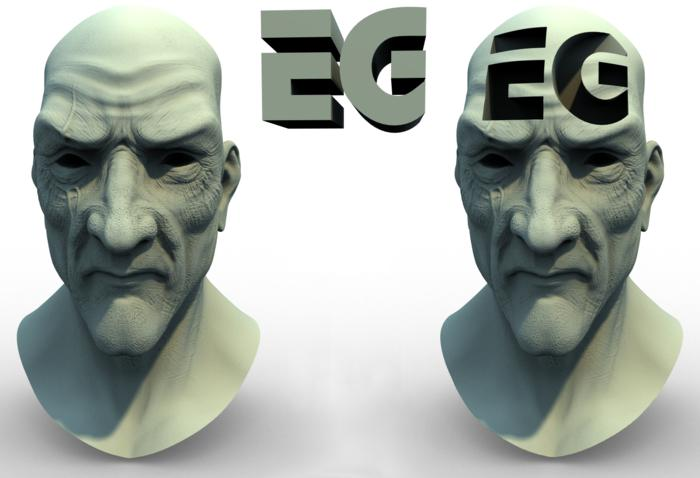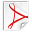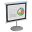# Wavelet Rasterization

This paper won the Best Paper Award at Eurographics 2011. A method for quickly calculating analytically box-filtered rasterizations of 2D polygons and 3D triangle meshes. Wavelets calculate a multiresolution image over a quadtree or octree that is robust to degenerate input.

Ammended: Corrected subscripts of equations in Appendix C and Figure 2.download preprint

## BibTeX

```@article{Manson:2011:WR,
author  = {Josiah Manson and
Scott Schaefer},
title   = {Wavelet Rasterization},
journal = {Computer Graphics Forum (Proceedings of Eurographics)},
year    = {2011},
volume  = {30},
number  = {2},
pages   = {395--404},
}
```

## Abstract

We present a method for analytically calculating an anti-aliased rasterization of arbitrary polygons or fonts bounded by Bezier curves in 2D as well as oriented triangle meshes in 3D. Our algorithm rasterizes multiple resolutions simultaneously using a hierarchical wavelet representation and is robust to degenerate inputs. We show that using the simplest wavelet, the Haar basis, is equivalent to performing a box-filter to the rasterized image. Because we evaluate wavelet coefficients through line integrals in 2D, we are able to derive analytic solutions for polygons that have Bezier curve boundaries of any order, and we provide solutions for quadratic and cubic curves. In 3D, we compute the wavelet coefficients through analytic surface integrals over triangle meshes and show how to do so in a computationally efficient manner.

## Supplementaltris2indicator.zip

This is an implementation of rasterizing a 3D triangle mesh into an octree of wavelet coefficients, from which a regular grid of function values is easily created. A small modification to the code can easily calculate function values over an octree also. The OBJ input format is somewhat restrictive, but the parser is blazing fast.

August 2, 2011: Can now load obj files with normals and texture coordinates, added some documentation, added an executable, and performed some bug fixes.wavelet_raster.zip

This is an implementation of rasterizing a 2D polygon or collection of Bezier curves. FreeType is used to extract curves from font files and as a comparison renderer. I have included a TrueType font, Vladimir, for convenience. The font comparison figure in the paper is the upper case 'T' of this type face.presentation_EG2011.pptx

This is the set of slides that I used in my presentation of the paper at Eurographics 2011.